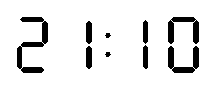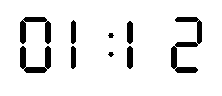### Problem Statement

We have a big digital wall clock, but while hanging it on the wall, we mounted it upside-down by mistake (i.e. the display was rotated 180 degrees). To make things worse, the clock has a complicated mechanism inside that makes it go slow when it is upside-down, needing secsPerMinute seconds to advance a minute instead of 60 seconds. Note that even at the moment we hang the clock on the wall, this is not necessarily set at the correct time. The clock itself only displays hours (from 00 to 23) and minutes (from 00 to 59), including any leading zeros.

You are given a String currentTime in the form "HH:MM", representing the actual (correct) time at which the clock is mounted on the wall, and a String clockTime also in the form "HH:MM", representing the time the clock is displaying when it is mounted on the wall (the time that we would see if the clock was mounted normally, not upside-down). Assume in both times that the seconds part is 0, i.e., the time just changed to currentTime and the clock just advanced to clockTime. You are to compute the first time after the clock is mounted on the wall that the clock shows the correct time (i.e., the display as shown now that the clock is mounted upside-down represents the correct time) and return this as a String in the form "HH:MM", including any leading zeros. If the clock never shows the correct time, return an empty String.

When the clock time is read upside-down, the digits 0, 1, 2, 5 and 8 are the same, 6 is shown as 9 and 9 is shown as 6. The digits 3, 4 and 7 do not show any meaningful digits when read upside-down.

### Definition

 Class: SlowDigitalClock Method: firstCorrectTime Parameters: String, String, int Returns: String Method signature: String firstCorrectTime(String currentTime, String clockTime, int secsPerMinute) (be sure your method is public)

### Notes

-You may assume that when the clock display is updated to the next minute, this update takes zero time.

### Constraints

-currentTime and clockTime will have exactly 5 characters each, in the form "HH:MM". "HH" will be an integer between 00 and 23, inclusive (including any leading zeros), "MM" will be an integer between 00 and 59, inclusive (including any leading zeros).
-secsPerMinute will be between 61 and 1000, inclusive.

### Examples

0)

 `"01:11"` `"21:09"` `61`
`Returns: "01:12"`
 At the beginning, the clock shows the upside-down time "60:12" (of course, this is not a valid time, so this can not be the correct time). After 61 seconds, the clock will advance to 21:10, which is read upside-down as: 01:12. Since the correct time at the beginning was 01:11:00, now (61 seconds later) the time is 01:12:01, so (ignoring the seconds) the clock shows the correct time "01:12". The figure below shows the normal clock display when it shows 21:10.The figure below shows the same display upside-down (now showing 01:12).1)

 `"01:10"` `"21:09"` `61`
`Returns: "01:12"`
 In 120 seconds, the time is 01:12, but the clock still shows 21:10 (which upside-down reads 01:12).
2)

 `"12:50"` `"05:21"` `125`
`Returns: "12:50"`
 The clock already shows the correct time at the beginning (and this remains correct for the next 60 seconds), so currentTime is returned.
3)

 `"05:46"` `"23:50"` `240`
`Returns: "11:10"`
 Be careful when changing days (after 23:59 comes 00:00).
4)

 `"12:34"` `"23:45"` `197`
`Returns: "02:11"`
 The first time that the clock displays the correct time may occur after several days.
5)

 `"12:34"` `"23:45"` `300`
`Returns: ""`
 In this case, the clock never shows the correct time.
6)

 `"00:00"` `"00:01"` `86`
`Returns: "01:22"`

#### Problem url:

http://www.topcoder.com/stat?c=problem_statement&pm=4765

#### Problem stats url:

http://www.topcoder.com/tc?module=ProblemDetail&rd=7994&pm=4765

gepa

#### Testers:

PabloGilberto , lbackstrom , brett1479 , Olexiy

#### Problem categories:

Recursion, Simple Search, Iteration, Simulation, String Parsing